# Graph Types and Applications

Prerequisite: Graph Theory Basics – Set 1, Graph Theory Basics – Set 2

A graph G = (V, E) consists of a set of vertices V = { V1, V2, . . . } and set of edges E = { E1, E2, . . . }. The set of unordered pairs of distinct vertices whose elements are called edges of graph G such that each edge is identified with an unordered pair (Vi, Vj) of vertices.
The vertices (Vi, Vj) are said to be adjacent if there is an edge Ek which is associated to Vi and Vj. In such a case Vi and Vj are called end points and the edge Ek is said to be connect/joint of Vi and Vj.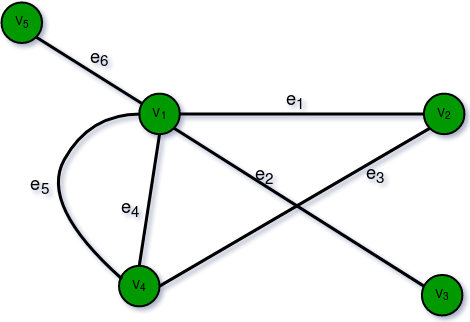Types of Graph:

• Finite Graphs: A graph is said to be finite if it has finite number of vertices and finite number of edges.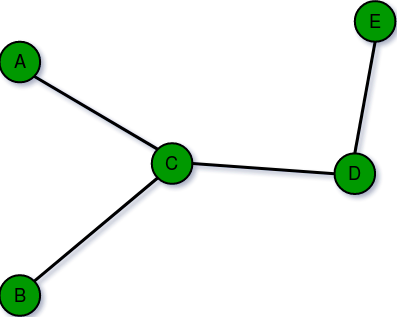• Infinite Graph: A graph is said to be infinite if it has infinite number of vertices as well as infinite number of edges.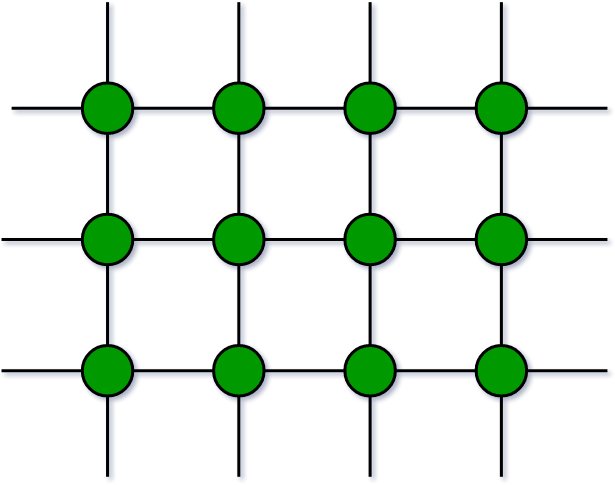• Trivial Graph: A graph is said to be trivial if a finite graph contains only one vertex and no edge.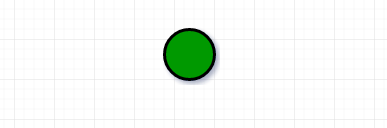• Simple Graph: A simple graph is a graph which does not contains more than one edge between the pair of vertices. A simple railway tracks connecting different cities is an example of simple graph.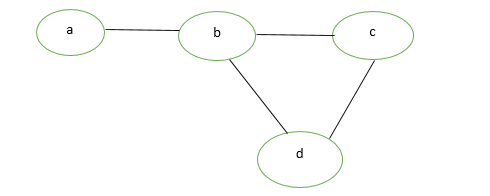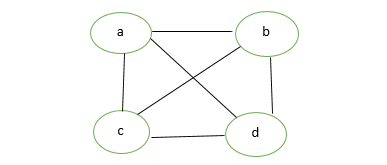• Multi Graph: Any graph which contain some parallel edges but doesn’t contain any self-loop is called multi graph. For example A Road Map.• Parallel Edges: If two vertices are connected with more than one edge than such edges are called parallel edges that is many roots but one destination.
• Loop: An edge of a graph which join a vertex to itself is called loop or a self-loop.
• Null Graph: A graph of order n and size zero that is a graph which contain n number of vertices but do not contain any edge.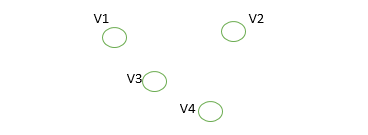• Complete Graph: A simple graph with n vertices is called a complete graph if the degree of each vertex is n-1, that is, one vertex is attach with n-1 edges. A complete graph is also called Full Graph.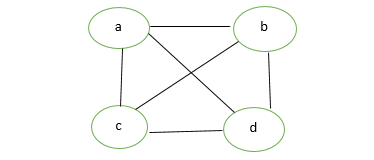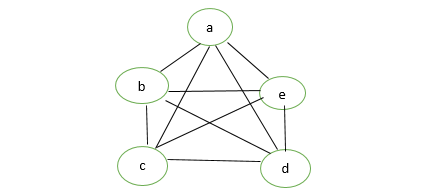• Pseudo Graph: A graph G with a self loop and some multiple edges is called pseudo graph.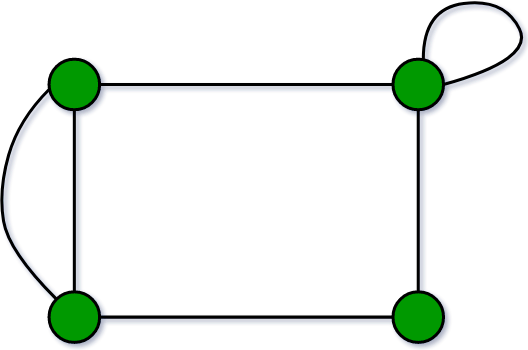• Regular Graph: A simple graph is said to be regular if all vertices of a graph G are of equal degree. All complete graphs are regular but vice versa is not possible.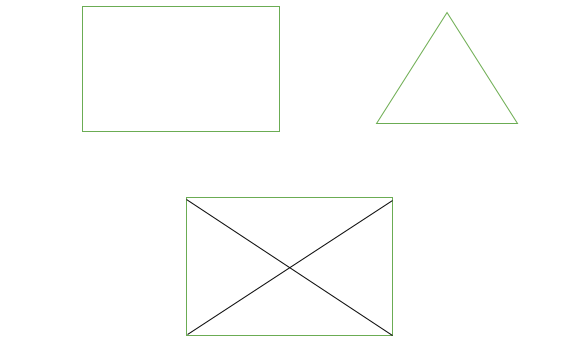• Bipartite Graph: A graph G = (V, E) is said to be bipartite graph if its vertex set V(G) can be partitioned into two non-empty disjoint subsets. V1(G) and V2(G) in such a way that each edge e of E(G) has its one end in V1(G) and other end in V2(G).
The partition V1 U V2 = V is called Bipartite of G.
Here in the figure:
V1(G)={V5, V4, V3}
V2(G)={V1, V2}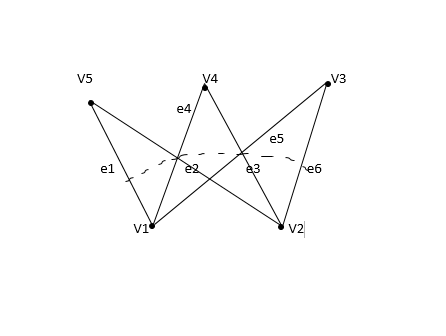• Labelled Graph: If the vertices and edges of a graph are labelled with name, data or weight then it is called labelled graph. It is also called Weighted Graph.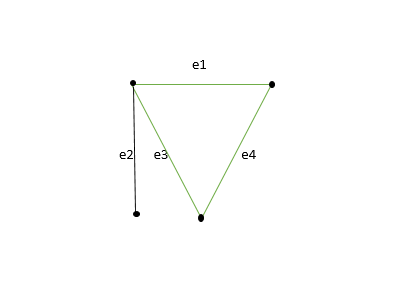• Digraph Graph: A graph G = (V, E) with a mapping f such that every edge maps onto some ordered pair of vertices (Vi, Vj) is called Digraph. It is also called Directed Graph. Ordered pair (Vi, Vj) means an edge between Vi and Vj with an arrow directed from Vi to Vj.
Here in the figure:
e1 = (V1, V2)
e2 = (V2, V3)
e4 = (V2, V4)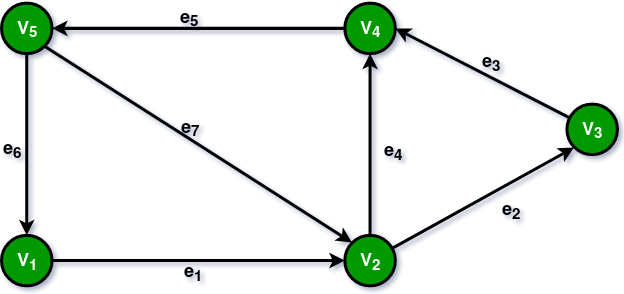• Subgraph: A graph G = (V1, E1) is called subgraph of a graph G(V, E) if V1(G) is a subset of V(G) and E1(G) is a subset of E(G) such that each edge of G1 has same end vertices as in G.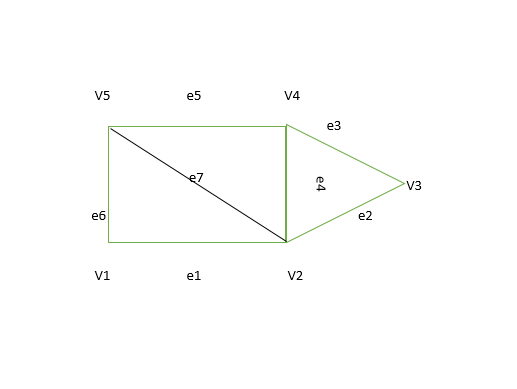Types of Subgraph:

• Vertex disjoint subgraph: Any two graph G1 = (V1, E1) and G2 = (V2, E2) are said to be vertex disjoint of a graph G = (V, E) if V1(G1) intersection V2(G2) = null. In figure there is no common vertex between G1 and G2.
• Edge disjoint subgraph: A subgraph is said to be edge disjoint if E1(G1) intersection E2(G2) = null. In figure there is no common edge between G1 and G2.

Note: Edge disjoint subgraph may have vertices in common but vertex disjoint graph cannot have common edge, so vertex disjoint subgraph will always be an edge disjoint subgraph.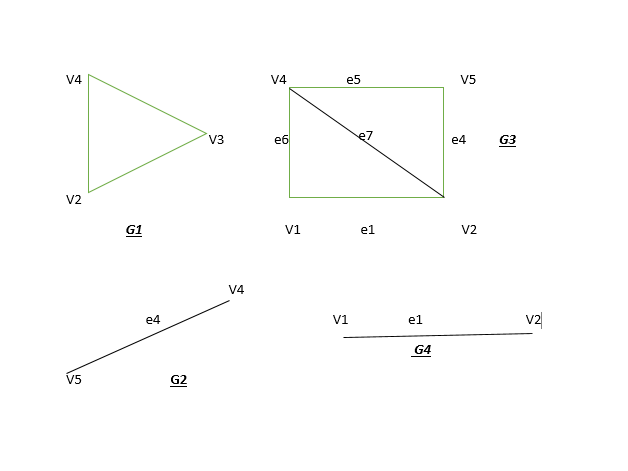• Connected or Disconnected Graph: A graph G is said to be connected if for any pair of vertices (Vi, Vj) of a graph G are reachable from one another. Or a graph is said to be connected if there exist atleast one path between each and every pair of vertices in graph G, otherwise it is disconnected. A null graph with n vertices is disconnected graph consisting of n components. Each component consist of one vertex and no edge.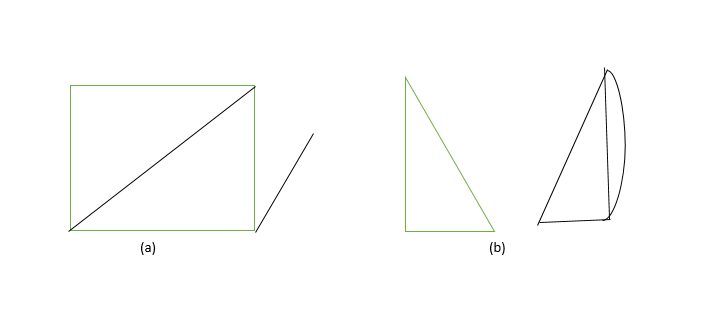• Cyclic Graph: A graph G consisting of n vertices and n> = 3 that is V1, V2, V3- – – – – – – – Vn and edges (V1, V2), (V2, V3), (V3, V4)- – – – – – – – — -(Vn, V1) are called cyclic graph.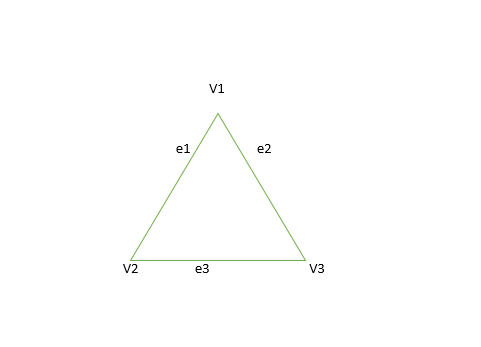• Application of Graphs:

• Computer Science: In computer science, graph is used to represent networks of communication, data organization, computational devices etc.
• Physics and Chemistry: Graph theory is also used to study molecules in chemistry and physics.
• Social Science: Graph theory is also widely used in sociology.
• Mathematics: In this, graphs are useful in geometry and certain parts of topology such as knot theory.
• Biology: Graph theory is useful in biology and conservation efforts.

Attention reader! Don’t stop learning now. Get hold of all the important DSA concepts with the DSA Self Paced Course at a student-friendly price and become industry ready.

My Personal Notes arrow_drop_upCheck out this Author's contributed articles.

If you like GeeksforGeeks and would like to contribute, you can also write an article using contribute.geeksforgeeks.org or mail your article to contribute@geeksforgeeks.org. See your article appearing on the GeeksforGeeks main page and help other Geeks.

Please Improve this article if you find anything incorrect by clicking on the "Improve Article" button below.

Article Tags :
Practice Tags :

8

Please write to us at contribute@geeksforgeeks.org to report any issue with the above content.